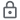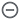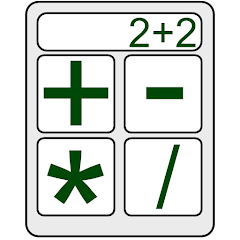# Tabbed Calculator

500+Everyone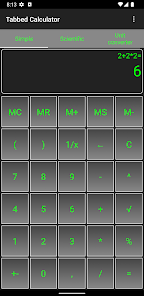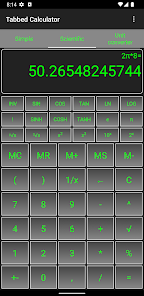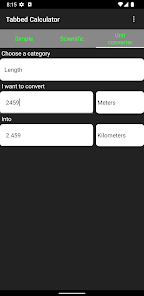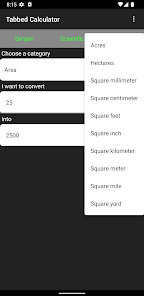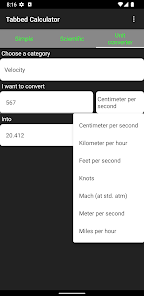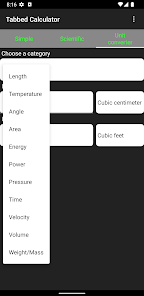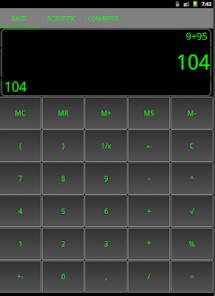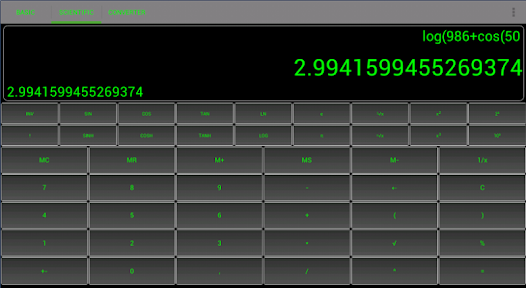Tabbed Calculator is an application that contains basic, scientific calculator and unit converter each in separate tab.

Each tab have own memory so You can two indepentent calculations in basic and scientific part.
of app.

You can convert units like time, volume, areas, angles, length and much more!

In scientific calculator "e" means Euler number (2.718281828459045....).

Of course order of evaluations is preserved.

Main features:
-basic, scientific calculator
-unit converet which can convert: angles, lenghts, time, areas, power and more
-support for percentages calculating (10+10% = 11 and 10*50% = 5)
-preserves the order of evaluations (2+2*2 = 6)
-contains π and Euler number( "e" at scientific calculator)
-simple design
Updated on
Jan 15, 2022

## Data safety

Safety starts with understanding how developers collect and share your data. Data privacy and security practices may vary based on your use, region, and age. The developer provided this information and may update it over time.No data shared with third parties